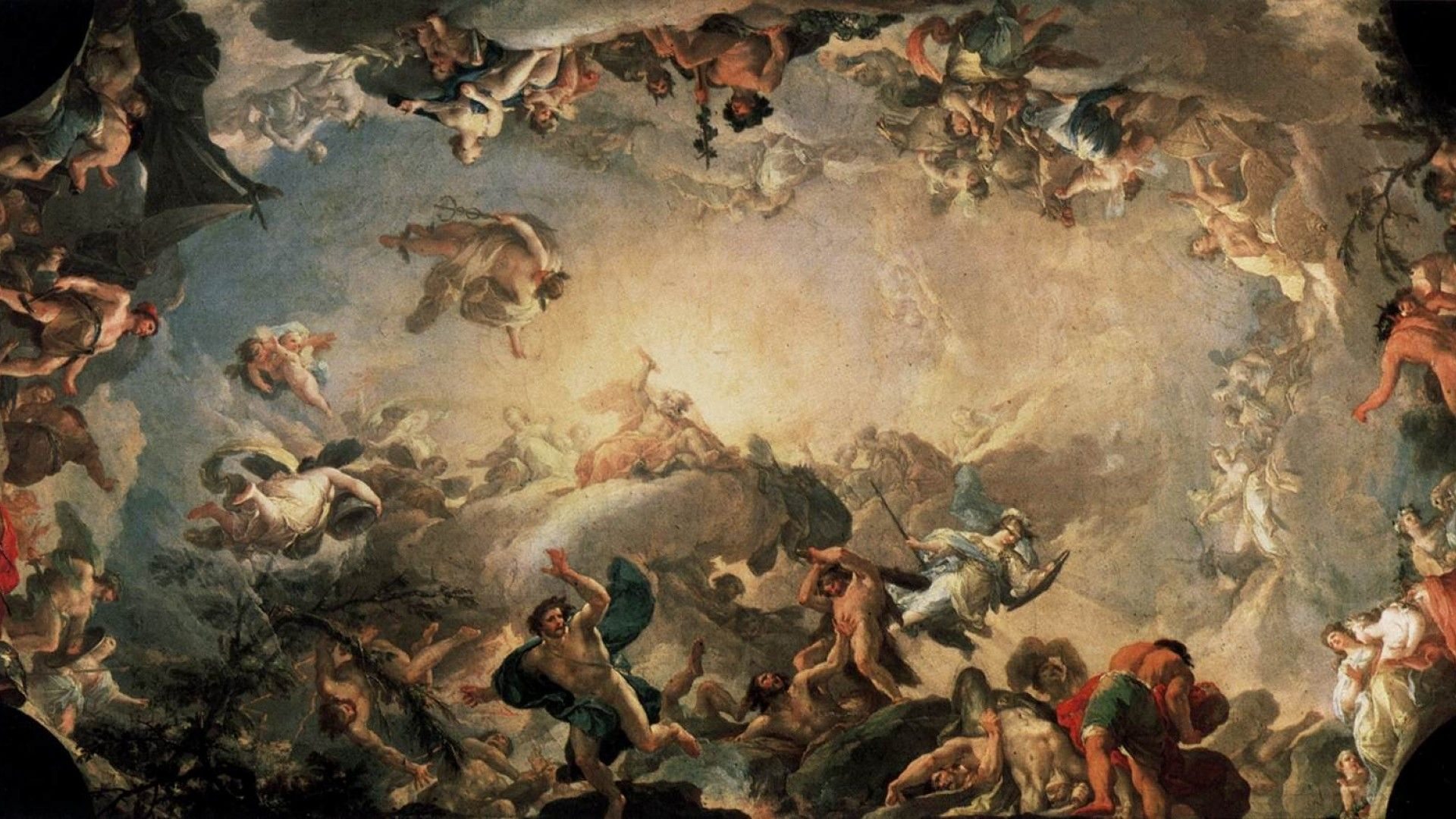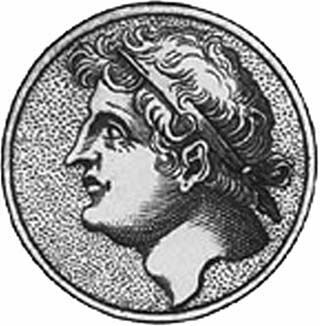TheDomeENTER THE WORLD OF MYTHS AND LEGENDS## NicomedesNicomedes (Greek: Νικομήδης; c. 280 – c. 210 BC) was an ancient Greek mathematician.

Nothing is known of the life of Nicomedes. His period of activity can be only approximately inferred from the facts that he criticized the solution of Eratosthenes (fl. 250 B.C.) to the problem of doubling the cube and that Apollonius (fl. 200 B.C.) named a curve “sister of the cochlioid,” presumably as a compliment to Nicomedes, who had discovered the curve known as cochlioid, cochloid, or conchoid.1 The second inference is far from secure, but what we know of Nicomedes’ mathematical investigations fits well into the period of Archimedes (d. 212 B.C.).

The work for which Nicomedes became famous was called On Conchoid Lines2. We know it only through secondhand references. In it Nicomedes described the generation of a curve, which he called the “first conchoid,” as follows (see Figure 1): Given a fixed straight line AB (the “canon”) and a fixed point E (the “pole”), draw EDG perpendicular to AB, cutting it at), and make DG a fixed length (the “interval”); then let GDE move about E in such a way that D is always on AB (thus when D reaches T), G will have reached T). G will then describe a curve, LGTM, the first conchoid. The advantages of this curve are that it is very easy to construct (Nicomedes described a mechanical instrument for drawing it)3 and that it can be used to solve a variety of problems, including the “classical” problems of doubling the cube and trisecting the angle. These are all soluble by means of the auxiliary construction which we may call the “lemma of Nicomedes”: Given two straight lines, X,Y, meeting in a given angle and a point P outside the angle, it is possible to draw a line through P cutting X and Y so

that a given length, l, is intercepted between X and Y. This is done by constructing a conchoid with “canon” X, “pole” P, and “interval” l; the intersection of this conchoid and Y gives the solution.

Nicomedes solved the problem of finding two mean proportionals (to which earlier Greek mathematicians had reduced the problem of doubling the cube) as shown in Figure 2.

Given two straight lines AB, BG, between which it is required to find two mean proportionals. Complete the rectangle ABGL. Bisect AB, BG in D and E, respectively. Join LD and produce it to meet GB produced in H Draw EZ perpendicular to EG, of length such that GZ = AD. Join HZ, and draw GT parallel to it. Then draw ZTK, meeting BG produced in K, so that TK = AD (this is possible by the “lemma of Nicomedes”), Join KL and produce it to meet BA produced in M. Then

and GK, MA are the required mean proportionals.4

Nicomedes also showed how to trisect the angle by means of his lemma and proved that the “first conchoid” is asymptotic to its “canon.”5 In addition he described what he called the “second, third, and fourth conchoids” and their uses. The ancient sources tell us nothing about them beyond their names, but it has been plausibly conjectured that they are to be identified with the other branch of the curve in its three possible forms. In modern terms the curve is a quartic whose equation is, in polar coordinates,

or, in Cartesian coordinates,

(x-a)2 (x2+y2)-l2x2=0.

This curve has two branches, both asymptotic to the line x = a. The lower branch (see Figure 1, in the lower part of which the three forms are depicted) has a double point at the pole E which is either a node, a cusp, or an isolated point according as l ⋛ a (here l corresponds to the interval, a to the distance from the pole to the canon). This second branch can be constructed in the same way that Nicomedes constructed the first, with the sole difference that the interval is taken on the same side of the canon AB as the pole E.6

As far as is known, all applications of the conchoid made in antiquity were developed by Nicomedes himself. It was not until the late sixteenth century, when the works of Pappus and Eutocius describing the curve became generally known, that interest in it revived and new applications and properties were discovered. Viète used the “lemma of Nicomedes” as a postulate in his Supplementum geometriae (1593) to solve a number of problems leading to equations of the third and fourth degrees, including the construction of the regular heptagon. Johann Molther, in his little-known but remarkable Problema deliacum (1619), used the same lemma for an elegant reworking of the old problem of finding two mean proportionals. The conchoid attracted the attention of some of the best mathematicians of the seventeenth century. Descartes discussed the construction of tangents to it (in his Géométrie of 1637); Fermat and Roberval treated the same problem with respect to both branches. Huygens discovered a neat construction of the point of inflection (1653). Newton discussed the curve more than once, and in his Arithmetica universalis he recommended its use as an auxiliary in geometry because of the ease of its construction (it is in fact sufficient to solve any problem involving equations of the third and fourth degrees). In the appendix to the Arithmetica, on the linear construction of equations, he makes extensive use of the “lemma of Nicomedes.” The seventeenth century also saw the first generalization of the conchoid, produced by taking a circle instead of a straight line for canon: this generates Pascal’s limaçon.

Notes

1. Eutocius, Commentary on Archimedes’ Sphere and Cylinder, in Archimedis Opera omnia, J. L. Heiberg, ed., Ill, 98; Simplicius, Commentary on Aristotle’s Categories Kalb-fleisch, ed., p. 192.

2. Eutocius, loc. Cit. It is uncertain whether Nicomedes called the curve κοχλο∊ιδής, κοχλο∊ιδής, or κογχο∊ιδής (all three are found in our sources). The first two mean “snail-shaped”; the third, “mussel-shaped.”

3. Ibid.

4. For a proof see Pappus, Synagoge, bk. 4, sec. 43, Hultsch, ed., I, 248–250, repro. in Heath, History of Greek Mathematics, I, 260–262. In fact all of the construction after the determination of point A’ is superfluous, for ZT= MA and therefore GK, ZT are the required mean proportionals. As D. T. Whiteside pointed out to me, this was realized by Molther (Problema deliacum, pp. 55–58), and by Newton Mathematical Papers, II, prob. 15, pp. 460–461).

5. On trisection of the angle see Pappus, Synagoge, bk. 4, sec. 62, Hultsch, ed., I, 274–276; see also Proclus. Commentary on Euclid I, Friedlein, ed., p. 272. On the “first conchoids” being asymptotic to its “canon,” see Eutocius, op. cit., in Archimedis Opera omnia, J. L. Heiberg, ed., Ill, 100–102.

6. I do not know who first proposed this identification of Nicomedes’ “second, third, and fourth conchoids,” but a probable guess is either Fermat or Roberval. In a letter to Roberval of 1636, Fermat (Oeuvres, II, 94) mentions the “second conchoid of Nicomedes.” Roberval refers to the two branches as “conchoide de dessus” and “conchoide dc dessous,“ respectively (“Composition des mouvemens,” in Ouvrages de mathçmatique[17.11], p. 28).

BIBLIOGRAPHY

The principal ancient passages concerning Nicomedes are Pappus, Synagoge, F. Hultsch, ed., I (Berlin, 1875), bk. 3, sec. 21, p. 56; bk. 4, sees. 39–45, pp. 242–252, and sees. 62–64, pp. 274–276; Eutocius, Commentary on Archimedes’ Sphere and Cylinder, in Archimedis Opera omnia. J. L. Heiberg ed 2nd ed., III (Leipzig, 1915), 98–106; Proclus, Commentary on Euclid I G. Friedlein, ed. (Leipzig, 1873), 272; and Simplicity Commentary on Aristotle’s Categories, K. Kalbfleisch, ed., which is Commentaria in Aristotelem Graeca, VIII (Berlin, 1907), 192. The best modern account of Nicomedes is Gino Loria, Le scienze esatte nell’antica Grecia,2nd ed. (Milan, 1914), 404–410. See also T. L. Heath, A History of Greek Mathematics(Oxford, 1921), I, 238–240, 260 262, and II, 199. There is no adequate account of the treatment of the conchoid in the sixteenth and seventeenth centuries. The best available is Gino Loria, Spezielle algehraische und transzendente ebene Kurven, 2nd ed. (Leipzig-Berlin, 1910), I, 136–142, which also gives a good description of the mathematical properties of the curve; see also F. Gomes Teixeira, Traité des courbes spéciales remarquables, I, which is vol. IV of his Obras sobre matematica (Coimbra, 1908), 259–268.

On generalizations of the conchoid see Loria, Spezielle… Kurven, pp. 143–152. Viète’s Supplementum geometriae is printed in his Opera mathematica, F. van Schooten, ed. (Leiden, 1646; repr. Hildesheim, 1970), 240–257. Johann Molthcr’s extremely rare opuscule, Problema deliacum de cubi duplicatione, was printed at Frankfurt in 1619. For Descartes’s treatment of the conchoid see The Geometry of Rene Descartes, trans. by D. E. Smith and M. L. Latham (Chicago-London, 1925), 113–114. The discussions of Fermat and Roberval are printed in Pierre de Fermat, Oeuvres, P. Tannery and C. H. Henry, eds., II (Paris, 1894), 72, 82, 86–87. See also Roberval, Ouvrages de mathématique (The Hague, 1731), 28–32 (on Pascal’s limacon, see p. 35). Huygens’ solution is printed in Oeuvres complètes de Christiaan Huygens, XII (The Hague, 1910), 83–86. For Newton’s treatment see The Mathematical Papers of Isaac Newton, D. T. Whiteside, ed., II (Cambridge, 1968), prob. 15, pp. 460–461, and especially the app. to his “Universal Arithmetick,” printed in The Mathematical Works of Isaac Newton, D. T. Whiteside, ed., II (New York-London, 1967), 118–134.



### Sources

 "Encyclopedia.com" by G. J. Toomer

 "For "Conchoid of Nicomedes" (Click Here)" by MathWorld - Weisstein, Eric W.

### Our Mobile Application

Check out Our Mobile Application "Ancient Greece Reloaded"